# Mathematical Digression: Euler-Lagrange Equation

Suppose we want to minimize the function: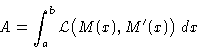(4)
with M' =dM/dx, and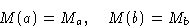(This is called Lagrange problem).

Standard calculus: if we want to minimize y(x), we consider a small deviation: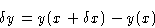At the minimum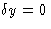In this situation we have a functional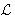that depends on the function M(x). Same idea: consider a deviation: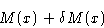and calculate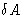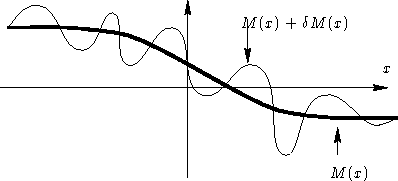We have: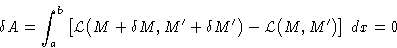Expansion: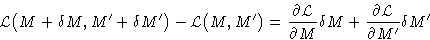and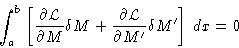Second term: integrating by parts: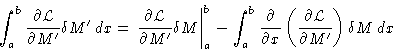Result: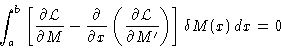This is possible only if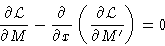(5)
This is Euler-Lagrange equation, well known in Classical Mechanics.

© 1997 Boris Veytsman and Michael Kotelyanskii
Thu Oct 16 20:58:44 EDT 1997